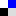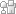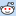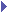Posted by kyle on June 25, 2006 11:56 PM bookmark / share:### Force Directed Graphs, Performance, and Trees?

This weekend I finally got a chance to go over the wishlist that has been accumulating for the Force Directed Graph libraries I posted about earlier this month. Nearly everyone has asked that I do something about the performance problems, and I have some good news. If you're new to this discussion, check out the earlier postings and comments:

First, a recap

Let's talk briefly about why Force Directed Graph algorithms don't scale very well.

Each node in a graph behaves like a "particle" in space. Some particles have attractive forces between them. These are usually represented as edges on the graph. Every particle has repulsive forces between itself and every other particle. These are the forces that make nodes "avoid" each other while the graph organizes. The combination of these forces on a given node help dictate a new position for the node. Any time a node has changed position, the forces it places on all of the other nodes have changed, so we have to reculate their positions.

Attractive forces are no problem to calculate because the number of edges in a graph is normally relatively small compared to the number of nodes. The repulsive forces are the painful part, but a critical part of what makes these graphs neat to look at. The calculation of the repulsive force acting on a node at any given time has On2 complexity. If this is a new concept, this means that the number of calculations performed doubles for every node added to the graph. Optimizing such algorithms is difficult.

I like Force Directed Graphs, but there will always be a scaling problem. I noticed that a lot of the apps people have submitted to me via comments and e-mails model tree structures and might not need all of the features of a Force Directed Graph. Even Visual Wordnet is really a tree model. So over Saturday morning cartoons I decided to tackle Tree Graphs. In my implementation of these graphs, each node is only aware of its own children, so calculations are much less complex, but it's possible for nodes from disperate branches to collide.

Some prototypes have emerged. I'm trying to merge in a few concepts from the world of Force Directed Graphs to make Tree Graphs more visually appealing. Understand these examples aren't yet fully interactive, but they'll give you a sneak peak at the speed improvement.Example #1Example #2 (incrementally builds a random tree with 50 or so nodes.)Example #3 (see comments ... first whack at distribution correction (added 6.27))

I hope you can see some promise here. The end result should be a little faster due to some unexploited optimizations and will enable an author to sacrifice fluidity for speed when more drawing power is needed. Already, these scale a whole lot better than a Force Directed implementation.

Okay, I mocked that up too. Remember this is just an early prototype so it may look and act a little funny.Prototyping Visual Wordnet with Tree Graphs

Using this code for your applications

I hope to have time to make a more "official" version of the Force Directed and Tree Graph code that anyone can use as a library in applications. I've gotten a lot of great comments and suggestions that I will be addressing soon. In the meantime, I'm not going to let my busy schedule get in the way of your app, so if you're brave you can use this stuff right now. It's all covered under a Creative Commons Attribution License.

The critical code is in treegraph.js. See examples for usage.

Also, let me know what you think and what you're up to. It really drives where this stuff goes from here. Thanks!

Really slick! Can't wait to see where this goes.

Hey Kyle,

Awesome work!

You mentioned in your original Visual Wordnet that you might be planning an entropy-based throttle to prevent the CPU from spending cycles once the graph has settled. I was wondering if you've had a chance to look at this yet, or if not, if you wouldn't mind pointing me to where such code should go (specificaly in the tree graph version), as I'd be willing to take a swing at it myself and would gladly post my code here to share with others.

Cheers,
Mike

Hey Kyle,

One more thing. Is it possible to make the script(s) work with the XHTML Transitional DOCTYPE? If I add the following DOCTYPE declaration, all the nodes just sit in the upper-left corner:

Any info would be greatly appreciated!

Mike

Mike,

Thanks! Implementing a control to suspend animation when the graph settles will be super easy in the Tree Graph:

Every node has a 'position' vector and a 'target' vector. You can simply turn off the animation "thread" (call timer.stop()) when all of the nodes have reached their target (node['position']['x'] == node['target']['x'] && node['position']['y'] == node['target']['y']). You'll probably perform the check in the "setChildrenForces" method of TreeGraph and disable the timer from the update() method.

You may need to turn animation on again when there is activity like the addition of new nodes or mouse dragging (which I'll implement soon). To turn animation on again when a node is added, just call timer.start() from the addChild() method in TreeNode. You'll probably also want to create a flag to keep track of the state of the timer you don't start multiple "threads".

I hope that helps and look forward to seeing what you come up with. Thanks!

Mike, I haven't done any experiments with different DOCTYPE flavors. I'm sure there's a fix. I'll add this to the list.

Hey Kyle,

Got the animation suspension working in a primitive form. It seems that the position and target for most points will never be exactly equal, so a reasonable range around the target needs to be used instead. Going to keep tweaking it and will post what I have later today or (more likely) tomorrow.

As for the DOCTYPE issue, I've made some progress. It seems that for XHTML Transitional to work (and likely any XHTML DOCTYPE), you need to explicitly state the units of CSS properties (in this case, px). So, in any place where we set a CSS property for left, top, etc, we need to add a +'px' to the end. i.e.:

node['children'][i]['domNode'].style.left = node['children'][i]['position']['x']+'px';

I count 8 locations where this needs to be changed in treegraph.js.

Also, the px's need to be added to the Edge Point Template section as well:

pixTmpl.style.width = '2px';
pixTmpl.style.height = '2px';

This corrects the issues in FireFox, but IE does something weird and does not center the origin vertically in the page with an XHTML DOCTYPE declared. Still haven't figured out why this is, but I'm looking into it.

Mike

Great findings Mike!

This morning I added "Example #3". Since collisions are possible in these graphs, I want to minimize the liklihood that big branches will be neighbors. This is an early attempt to create a heterogeneous distribution of "branch weights". In the example, the branches at every level of the graph are redistributed every few seconds.

Hey Kyle,

Ok, so I didn't get much time to clean up the code yesterday, but figured I'd post it here as-is for anyone who was interested in using it. (I have a feeling it may not display correctly, so if you could take a look at the post and convert characters as necessary or post the file, that'd be great.)

---- START OF SCRIPT treegraph.js ----

//
// view a copy of this license, visit
// or send a letter to Creative Commons, 543 Howard Street, 5th Floor, San
// Francisco, California, 94105, USA.
//
// All copies and derivatives of this source must contain the license statement
// above and the following attribution:
//
// Author: Kyle Scholz http://kylescholz.com/
//

var TreeNode = function( id, radius, arc, x, y ) {
this['rootPos'] = 0;

this['id'] = id;

this['arc']=arc;

this['target']=new Object();
this['target']['x']=x;
this['target']['y']=y;

this['position']=new Object();
this['position']['x'] = FRAME_WIDTH/2;
this['position']['y'] = FRAME_HEIGHT/2;

this['force']=new Object();
this['force']['x'] = 0;
this['force']['y'] = 0;

this['domNode'] = document.createElement( "div" );
document.body.appendChild( this['domNode'] );
this['domNode'].style.position = "absolute";
this['domNode'].innerHTML = '';
this['domNode'].style.left = this['position']['x']+'px';
this['domNode'].style.top = this['position']['y']+'px';
this['domNode'].style.zIndex = 10;

this['children'] = new Array();

this.addChild = function( node ) {
this['children'].push(node);
this.updateChildren();
return node;
};

this.updateChildren = function() {
for ( var i=0; i var angle;
if ( this['children'].length == 1 ) {
angle=this['rootPos'];
} else {
angle=(this['arc']/(this['children'].length)*i) + this['rootPos'] - (this['arc']/(this['children'].length)*(this['children'].length-1))/2;
}
var node = this['children'][i];

node['rootPos'] = parseInt(angle);

var stagger = 0;
// if ( i % 2 ) { stagger = 8; }

node['target']['y'] = this['target']['y'] - (Math.cos(angle*(Math.PI/180)) * (this['radius']+stagger));
node['target']['x'] = this['target']['x'] - ((Math.sin(angle*(Math.PI/180)) * (this['radius']+stagger)))*graph['skew'];

var domNode = this['children'][i]['domNode'];
domNode.style.top = node['position']['y']+'px';
domNode.style.left = node['position']['x']+'px';

node.updateChildren();
}
};
}

// Distance:
var Distance = function(){};
Distance.prototype = {
calculate: function( pointA, pointB ) {
// X Distance
this['dx'] = pointA['x'] - pointB['x'];
// Y Distance
this['dy'] = pointA['y'] - pointB['y'];
this['d2'] = (this['dx']*this['dx']+this['dy']*this['dy']);
// Distance
this['d'] = Math.sqrt(this['d2']);
}
};

var TreeGraph = function( frame_width, frame_height, frame_top, frame_left ) {

this['frame_width']=frame_width;
this['frame_height']=frame_height;

this['frame_top']=frame_top;
this['frame_left']=frame_left;

this['skew']=frame_width/frame_height;

this['origin'];

this.setOrigin = function( origin ) {
this['origin'] = origin;
};

this.update = function() {
if (this.setChildrenForces(this['origin']) == 0) timer.stop();
};

this.setChildrenForces = function( node ) {
var moves = 0;
for( var i=0; i var distance = new Distance();
distance.calculate( node['children'][i]['target'], node['children'][i]['position'] );

if (node['children'][i]['position']['x'] node['children'][i]['position']['x'] > node['children'][i]['target']['x'] + 6 ||
node['children'][i]['position']['y'] node['children'][i]['position']['y'] > node['children'][i]['target']['y'] + 6) moves++;

this.attractiveForce( node, node['children'][i], distance );
node['children'][i]['position']['x'] += node['children'][i]['force']['x'];
node['children'][i]['position']['y'] += node['children'][i]['force']['y'];

node['children'][i]['domNode'].style.left = node['children'][i]['position']['x']+'px';
node['children'][i]['domNode'].style.top = node['children'][i]['position']['y']+'px';

node['children'][i]['force']['x'] = 0;
node['children'][i]['force']['y'] = 0;

moves += this.setChildrenForces( node['children'][i] );
this.drawEdge( node, node['children'][i] );
}
return moves;
};

// apply an attractive force between two nodes
this.attractiveForce = function( nodeI, nodeJ, distance ) {
var weight=5;
var attractive_force = (distance['d'] - weight)/weight;
if ( distance['dx'] ) {
nodeJ['force']['x'] += attractive_force * distance['dx'] / distance['d'];
}
if ( distance['dy'] ) {
nodeJ['force']['y'] += attractive_force * distance['dy'] / distance['d'];
}
};

this.drawEdge = function ( nodeI, nodeJ ) {

// edges should appear between center of nodes
var centeri = new Object();
centeri['x'] = nodeI['position']['x'] + 5;
centeri['y'] = nodeI['position']['y'] + 5;

var centerj = new Object();
centerj['x'] = nodeJ['position']['x'] + 5;
centerj['y'] = nodeJ['position']['y'] + 5;

// get a distance vector between nodes
var distance = new Distance();
distance.calculate( centeri, centerj );

// draw line
// k+factor at end determines dot frequency
var l = 6;
for ( var k=0; k var p = (distance['d'] / l) * k;
var pix;

try {
// dom updates are expensive ... recycle where we can
if ( !document.getElementById( 'edge' + nodeI.id + ':' + nodeJ.id ) ) {
var edge = document.createElement("div");
edge.id = 'edge'+nodeI.id+':'+nodeJ.id;
document.body.appendChild(edge);
}

if ( !document.getElementById('edge' + nodeI.id + ':' + nodeJ.id + ':' + k) ) {
pix = pixTmpl.cloneNode(true);
pix.id = 'edge' + nodeI.id + ':' + nodeJ.id + ':' + k;
document.getElementById('edge' + nodeI.id + ':' + nodeJ.id).appendChild(pix);
} else {
pix = document.getElementById('edge' + nodeI.id + ':' + nodeJ.id + ':' + k);
}
pix.style.left=centeri['x'] +(-1)*p*(distance['dx']/distance['d'])+'px';
pix.style.top=centeri['y'] +(-1)*p*(distance['dy']/distance['d'])+'px';
} catch ( e ) {

}
}
};

}

// Edge Point Template
var pixTmpl = document.createElement( 'div' );
pixTmpl.style.width = '2px';
pixTmpl.style.height = '2px';
pixTmpl.style.backgroundColor = '#888888';
pixTmpl.style.position = 'absolute';
pixTmpl.innerHTML="";

---- END OF SCRIPT treegraph.js ----

I chose a simple +/-6 range for the position around the target, which works well for the examples I tried. The timer stops near where movement of the nodes approaches zero. This range may need to be adjusted for certain applications of the code.

Also, for the IE issue with XHTML DOCTYPES, it seems IE requires that the height of the body be explicitly stated in the CSS. Once this is done, IE will properly calculate the FRAME_HEIGHT and center the graph. This does not appear to be an issue in FF, as FF is using the window height rather than body height.

I've also been trying to get the graph to display within a div tag on a page, rather that assume it is in its own window/page. I'm almost there, but for some reason, the edge points seem to be displaying significantly lower on the page, even when I've changed the containing element that the divs are appended to and setting all the position properties to "relative." Not sure why this is occurring, but I'll be working on it sometime today.

Mike

Mike,

Excellent! Your suggestions are a big help. I'm going to integrate your solution for suspending animation before the weekend. I made some big updates tonight to support heterogenous branch distribution and staggered node positions that increase readability. The Visual Wordnet example now takes advantage of these features. I haven't made any effort to clean up the code but it's all available. Thanks again! I hope you can share a URL for your project soon!

More great stuff!

Random ideas for optimizing the repulsion:
* do a proximity/collision detection, only do force-calcs for near neighbors (assuming the proximity compares are much easier than the full force-calcs)
* calculate repulsion at a lower resolution; eg only for one round-robined node per timer click -- smoothness would suffer but things might still eventually settle right
* maintain 2 sorted linked-lists of nodes in x and y; only calculate repulsion for nearest-neighbors in each axis (coudl be a win if maintaining the sort lists isn't too costly)

http://www.cricketschirping.com/weblog/?p=779

I combined your code with Walter Zorn's vector graphics library to draw edges as lines instead of dots:

```  drawEdge: function ( nodeI, nodeJ, distance ) {
var centeri = new Object();
centeri['x'] = this['frame_left'] + nodeI['position']['x'] + this.nodeRadius( nodeI );
centeri['y'] = this['frame_top'] + nodeI['position']['y'] + this.nodeRadius( nodeI );

var centerj = new Object();
centerj['x'] = this['frame_left'] + nodeJ['position']['x'] + this.nodeRadius( nodeJ );
centerj['y'] = this['frame_top'] + nodeJ['position']['y'] + this.nodeRadius( nodeJ );
if (jg) {
jg.drawLine(centeri.x, centeri.y, centerj.x, centerj.y);
}
}
```

It's not fast for lots of edges, so I wouldn't recommend replacing the dots in every situation but lines are certainly possible. Frankly I didn't vector lines would work at all (kind of like doing graph layout, for that matter. I'm impressed by both).

Thanks for taking the time to write this library btw.

Sean,

Sweet! This is really great! I'm a big fan of Walter Zorn's and this has been on my todo list. I promise a cleaner and more versatile release of these libararies is in the pipeline and these contributions will be part of it! Thanks!

I'm still blown away that any of this stuff works in the first place. I look forward to future updates.

Hey Kyle,

Sorry for the long absence. The recent 4th of July holiday and a new hire in the office kept me from working on this for a bit. I'm hoping to set aside some time in the next week to get the tree graph working in its own div tag and then should have some more impressive stuff to share.

Mike

Hey Mike,

Glad to hear you're still at it! I'm hacking away at a release candidate that will offer a cleaner and mostly common interface to both the tree and force directed graph libraries (and others in the pipeline). I plan to have this out in the next 2 weeks.

In the meantime, you can check out these updates:

- Added radial motion. This makes drawing a bit quicker but the most noticeable effect is that nodes don't travel through the center of the graph when a branch is moved.

- Made a marginally better heterogeneous distribution algortihm. This won't be part of the graph library itself, but I'll make it available as a helpful utility.

You can see the difference by looking at:

Example #3 with old features

then

Example #4 with new features